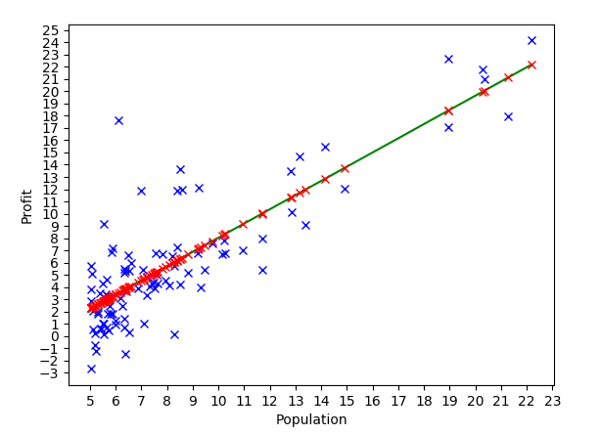Linear regression model created using TensorFlow.

### Introduction

As I described in my previous blog article Studying Machine Learning — First Impressions I decided to dedicate this winter’s studies in Machine learning. I have been doing the excellent Machine learning Coursera course and working with the exercises using Octave. This was basically the first step (theory) in my Machine learning study path.

Now that I finally after many weeks managed to walk through all material and exercises in that Coursera course I decided to do the same exercises using some industry level ML library (step two in my Machine learning study path). I evaluated the libraries and finally decided to start using TensorFlow. Why TensorFlow? TensorFlow seems to be one of the most used ML libraries. You can create the models using Python which makes the model creation pretty easy (using the Python REPL) if you are fluent in Python (see my previous Python Rocks! blog article). And the good side is that you can use the ML model (you created using Python) in Java, and therefore also in Clojure — my current choice of programming language (see more in my previous blog article Clojure Impressions Round Two).

### You Also Need Other Libraries When Creating ML Models…

When I was doing the first exercise Linear regression in TensorFlow I soon realized that you have to learn two other Python libraries as well:

• NumPy (scientific computing with Python) — without NumPy various matrix operations would be rather painfull, and ML is basically mostly rolling various matrices).
• Matplotlib (Python plotting library for creating graphics). It is important to visualize the data you are working with, and when working in Python Matplotlib is your friend. The graphic in the beginning of this blog article was generated using Matplotlib.

These libraries are not that difficult to learn, you can basically learn to use them on the fly when you are working with your ML model. And remember: Python REPL is your friend while learning any Python library.

### Python REPL

Let’s add a short Python REPL session example to visualize how you can use Python REPL to learn a new Python library and experiment with it before writing the actual code in your Python editor (by the way, my choice of Python IDE is excellent PyCharm).

``````>>> import src.ml_course_ex1a as ex1a
>>> model = ex1a.ProfitPopulationLinearRegression("ml_course_ex1a.ini", True)
>>> len(populations)
97
>>> import numpy as np
>>> populations_array = np.asarray(populations)
>>> populations_array
array([ 6.1101, 5.5277, 8.5186, 7.0032, 5.8598, ...
>>> X_train = np.asarray(populations)
>>> X_train_bias = model.appendBias(X_train)
>>> X_train.shape
(97,)
>>> X_train_bias.shape
(97, 2)
>>> X_train_bias
array([[ 1. , 6.1101],
[ 1. , 5.5277],
[ 1. , 8.5186],
``````

So, what’s happening here? You can import your source code in Python REPL and then interact with the code. Here I import the exercise module and instantiate the Linear regression class I created in the module. I use the readCsvFile method of that class just to get the data read into a Python tuple. Then I import NumPy library and experiment with it using the data.

Python REPL (read/eval/print/loop) is pretty good. It is much faster to use it to create production software in small blocks than e.g. traditional write/compile/deploy/test cycle used in Java. BUT… If you have never used Clojure REPL you know nothing about how powerfull a REPL can be…

### TensorFlow

The real reason for this blog article was TensorFlow so let’s focus on that. The code snippet below shows a typical TensorFlow usage. In TensorFlow you have tensors (matrices of various order) and operations which let tensors to flow from one step to another, hence name “TensorFlow”. Basically tensors and operations provide a higher level abstraction for using matrices and also auxiliary functions for various Machine learning algorithms.

``````X = tf.placeholder(tf.float32, [None, n])
y = tf.placeholder(tf.float32, [None, 1])
W = tf.Variable(tf.ones([n,1]), name="weights")
init = tf.global_variables_initializer()
y_prediction = tf.matmul(X, W) # As matrix multiplication in ex1.
# Cost
J = (1 / (2 * m)) * tf.reduce_sum(tf.pow(y_prediction - y, 2))
sess = tf.Session()
sess.run(init)# For recording cost history.
J_history = np.empty(shape=,dtype=float)
# Train iterations.
for i in range(iterations):
sess.run(step,feed_dict={X:X_train_normalized_bias, y:y_train})
J_history = np.append(J_history,sess.run(J,feed_dict={X:X_train_normalized_bias,y:y_train}))
``````

In the above code we create a couple of TensorFlow variables, and then define the y_prediction as matrix operation X * W (X = train set features, W = weights, or X * theta using Machine learning course terminology). Then we define the cost function as squared error (see in Coursera how prof. Ng describes it — the lectures were really good). Using TensorFlow to create a linear regression model like this is pretty straightforward once you have basic understanding of what linear regression is and how to get the weights (theta) using a gradient descent algorithm (here we use TensorFlow’s GradientDescentOptimizer method to do the heavy lifting; in the Coursera course exercises we did this part using matrix calculation— doing the same thing using basic matrix operations was amazingly simple in Octave).

If you are interested about the source code you can take a look in my Github repo in that exercise 1-ml-course-ex1 directory. The readme.md in that directory gives a more detailed explanation regarding the exercise. I plan to create more ML exercises using TensorFlow and I add the exercises to that directory when I have done them.

### Conclusions

I’m pretty sure this combination is going to be a killer knowledge for a future developer:

• Fluent in several programming languages.
• Experience to implement transaction intensive and parallel backend applications.
• Understanding and experience to create Big data systems.
• Knowledge to create Cloud infrastructure for your systems.
• Analytics and data science.
• Machine learning and Artificial intelligence. If you got interested I strongly recommend to start building your developer competence in that direction. You can start e.g. your Machine learning studies today — just sign in Coursera and start watching the first Machine Learning video lecture.